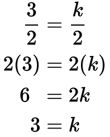# SAT Math Multiple Choice Question 311: Answer and Explanation

### Test Information

Question: 311

11.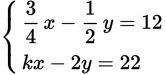If the system of linear equations above has no solution, and k is a constant, what is the value of k?

• A.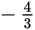• B.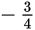• C. 3
• D. 4

Explanation:

C

Difficulty: Hard

Category: Heart of Algebra / Systems of Linear Equations

Strategic Advice: Graphically, a system of linear equations that has no solution indicates two parallel lines or, in other words, two lines that have the same slope. So, write each of the equations in slope-intercept form (y = mx + b) and set their slopes (m) equal to each other to solve for k. Before finding the slopes, multiply the top equation by 4 to make it easier to manipulate.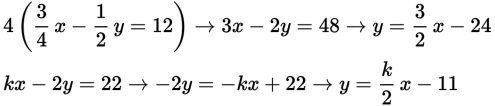The slope of the first line is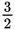, and the slope of the second line is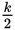. Set them equal and solve for k.